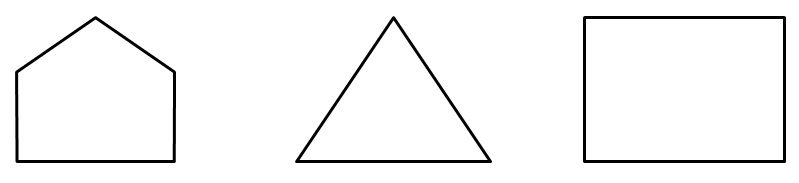Polygon is a simple closed figure made up of line segments.

So, Polygon is

1. Simple
2. Closed
3. Made up of line segmentsThey are all polygons.

1. Chapter 4 Class 6 Basic Geometrical Ideas
2. Concept wise
3. Curve, Polygons

Curve, Polygons

Chapter 4 Class 6 Basic Geometrical Ideas
Concept wise